# Memory usage from text concatenationWe know that2.02-04 Text Strings are bad for Anaplan models and that text functions and formulas using text are the slowest (slow in comparison to similarly-sized numeric formulas). One of the biggest contributing factors to the poor performance of text is the impact on the memory used by Anaplan.

The following diagrams show a comparison of Anaplan cell types to highlight the performance and memory use of text.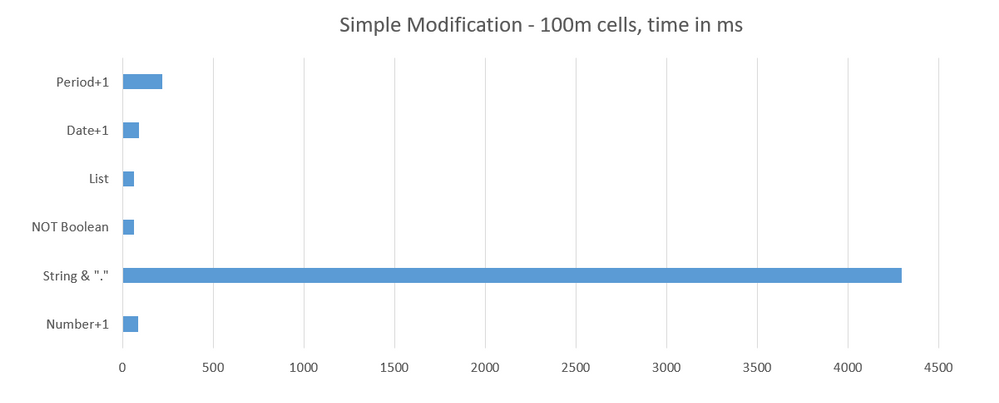Performance Cost by Cell type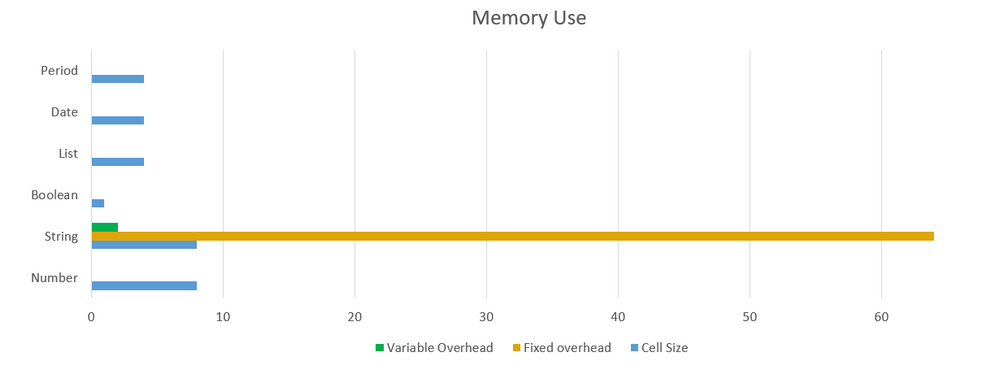Memory Use by Cell Type

This article will look at the memory used and created in joining text together—text concatenation.
If we look at a simple text concatenation that joins the codes from three lists to create a unique ID, here's the formula:

`CODE(ITEM(Product)) & "_" & CODE(ITEM(SKU)) & "_" & CODE(ITEM(Location))`

The 'Applies To' for this line item is "Product, SKU, Location"; and the lists have the following sizes 100, 2000, 10 items, respectively. This gives us a cell count of 2,000,000 cells.

This means we are doing four text additions 2 million times, and consequently, Anaplan is creating and throwing away millions of temporary strings in memory as it processes the formula.

Let's take a look at that process in detail. In the example below, we will use 1 byte to represent 1 character in the text; this is a basic simplification, the actual memory use will be higher.

The formula will first retrieve the Product code "123" and append an underscore to this text to create the first part of this string. The formula then retrieves the SKU code and appends that to the new part, creating another piece of text in memory. The first two bits of text we had in memory, "123" and "_", will be discarded and the memory will be cleared. We now have a new bit of text in memory "123_4567" and the previous parts can be discarded, 8 bytes in this case. This continues as the string is built up, new parts are created in memory and added to the previous parts to grow the string, the previous parts are then discarded and the memory cleared.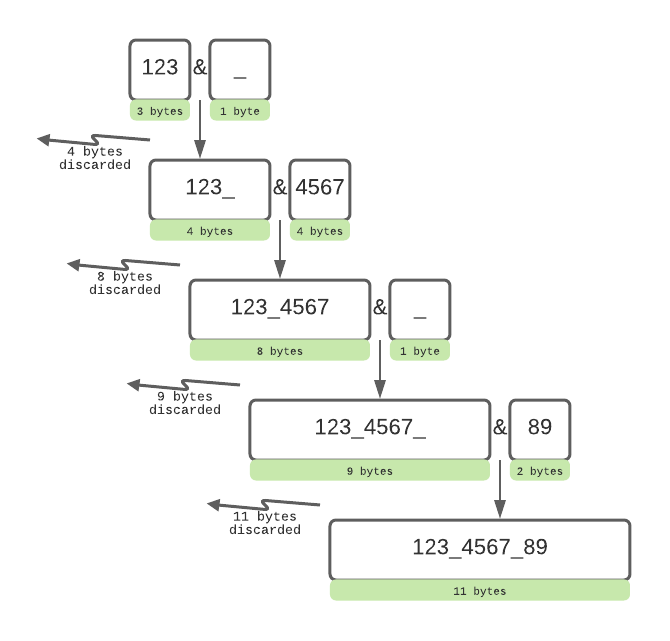It is this process of creating and discarding text parts that impact the memory and makes this formula slow. We use and clear a lot of memory in the construction of the final text, this is an expensive process in terms of computational power (relative to numeric calculations).

When there is a lot of this memory allocation happening at a fast rate, the processes of clearing up memory for the next calculations can cause delays, and in some circumstances "out of memory" errors. The process of memory clearing is known as "garbage collection", it will stop Anaplan and delay calculations while it is happening.

The charts below show memory use as concatenation increases, for all the charts the X-axis is the number of concatenations "&", 1 to 90.

The test model size is 704Mb, which remains constant as the length of the text in the cells increases with additional concatenation, or if the formula is set to BLANK; this is the estimated size Anaplan has calculated for this model based on the cell types.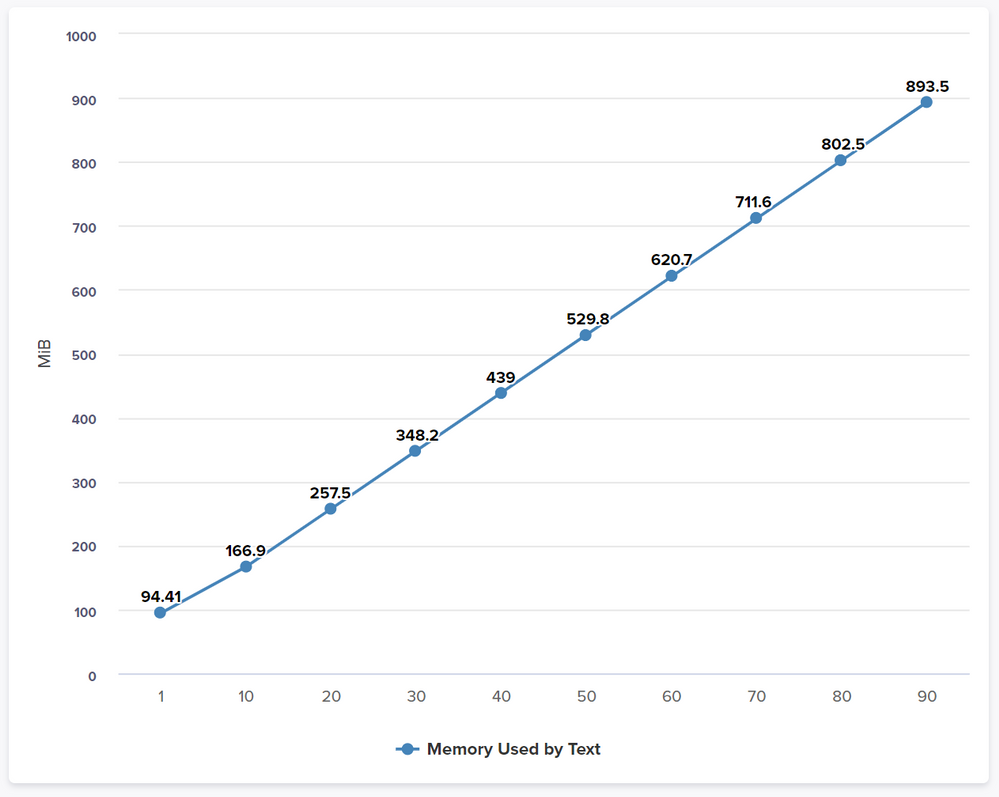Memory Used by Text

The graph above shows the actual memory used by the text line item; at 70 concatenations the memory from text has surpassed the predicted model memory size of 704Mb, from this point on the model will be using more memory than expected. As the concatenation increases the memory needed increases as the length of the text created grows, the length of each part of the text will also contribute to memory use; this example used numbers that varied from 1 to 4 digits, so quite short compared to the most text found in models.

As discussed above, the memory discarded in building the strings will also increase and to a much greater amount, the formula will create and discard a lot more memory than the final result.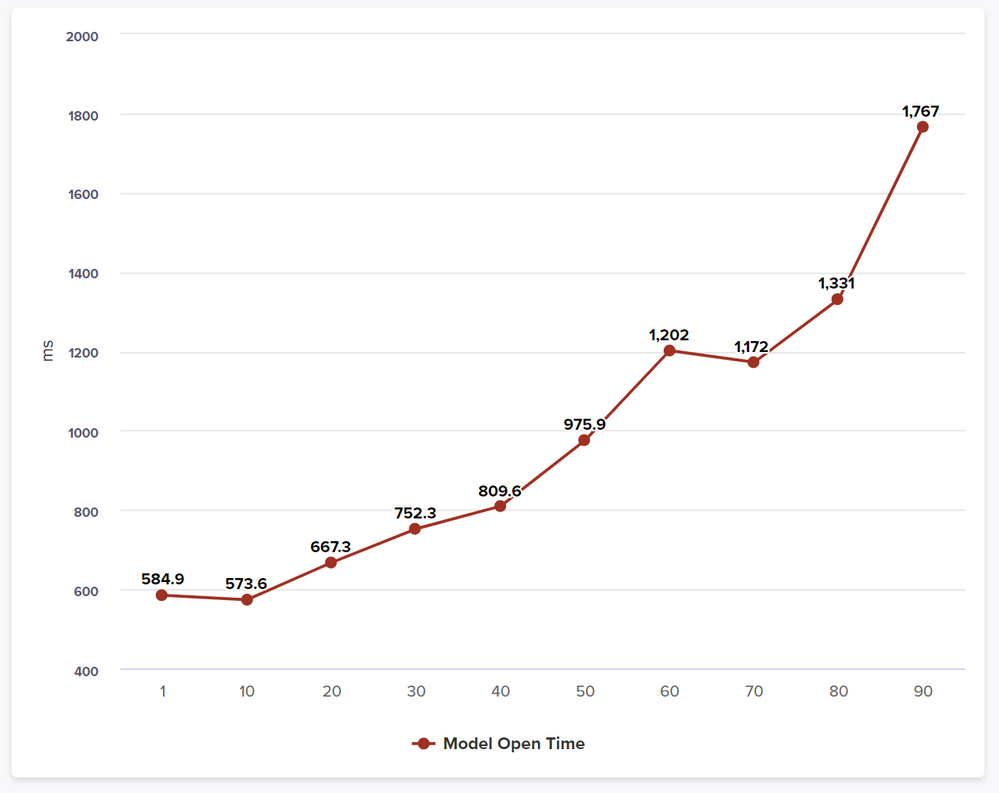Model Open Time

The performance will degrade as the concatenation increases; the increase in memory allocation and string manipulation will cause longer calculation times. We can see this in the chart above for the model open duration, where all line items are fully calculated. The model open durations increase at a higher rate than the memory growth, this is showing us that the impact of the concatenation is increasing and the delays from the garbage collection are growing longer at higher amounts of concatenation.

Similar effects are seen as cell count grows or text length increases, the larger the amounts of memory used and discarded the longer they take.

This performance hit would also apply to any calculations affecting this line item in the model. If a user added a new SKU to the list then the code would need to recalculate the whole code overall 2 million cells.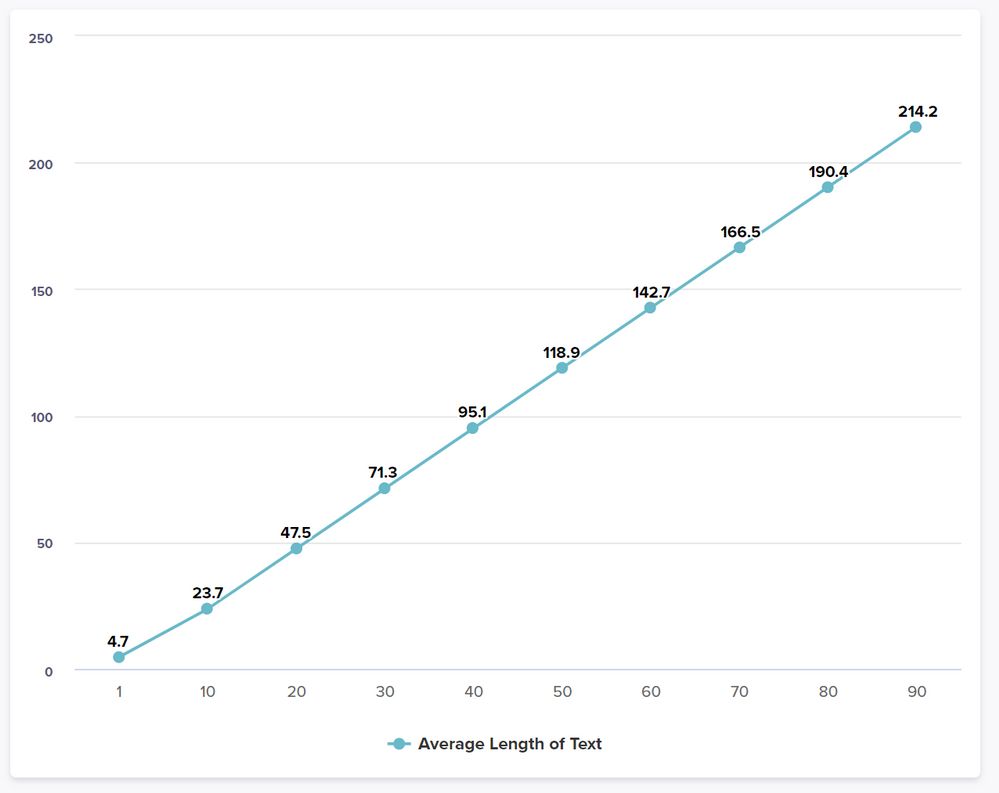Average Length of Text

The final chart just ties into the first in showing the linear growth of the text as concatenation increases. This is the average length of text across all cells (2 million in this test).

Avoiding memory problems with concatenation

This is covered by Planual rule2.02-05 Create "joins" in smallest hierarchy
Using our example formula above this would mean doing the following:

 Formula Applies To Cell Count Text1 = CODE(ITEM(Product)) & "_" Product 100 Text2 = "_" & CODE(ITEM(Location)) Location 10 Text3 = CODE(ITEM(SKU)) & Text2 SKU, Location 20,000 UniqueCode = Text1 & Text3 Product, SKU, Location 2,000,000

This shows how we can do just one concatenation at the full cell count; the bulk of the work is done at the lowest cell counts possible to minimize the performance and memory impact we discussed above. The extra text created is an impact on the model size but is a good trade-off for the increased performance and reduced memory use. This also has the benefit of separating the individual calculations, meaning that if we use the previous example of someone adding an SKU then Text1 and Text2 would not need to be recalculated, reducing the number of calculations needed to achieve the same result; this is Planual rule 2.02-18 Break up formulas

Let's look at some calculation timings for this formula optimization:

The original formula doing four concatenations took 1,089ms to calculate, and the model open took 0.19s. The model open for the optimized model was 0.07s. The model open time is reduced by 63%—the calculation cost is reduced by 68%.
This is the breakdown of the optimized calculation:

 UniqueCode 349.0ms Text3 3.542ms Text1 0.108ms Text2 0.060ms

If the order of the items was Product_Location_SKU then you would add both underscores this way:

`"_" & CODE(ITEM(Location)) & "_"`

This would then be how we do the most concatenations at the lowest cell count.

Also, be aware that the CODE(ITEM()) formulas should be in System modules and referenced, you can even create a single cell line item to reference the "_".

Text needs to be kept to a minimum where possible and line items should also keep concatenation to a minimum. I would suggest that if you have more than five concatenations, then think about why this text is needed and can this be achieved by alternative modeling. When using text for "error" messages, try to use booleans as well as list members for the error text for example.

Hopefully, this adds some detail and knowledge as to why the Planual rules were created and how important they are when considering model scalability. The memory used by our models is, unfortunately, something we need to consider with the current technology used by Anaplan, memory use is something all software has to consider. Following the Planual rules around the use of text and minimizing text joins will go a long way to helping in that goal.

Tagged: